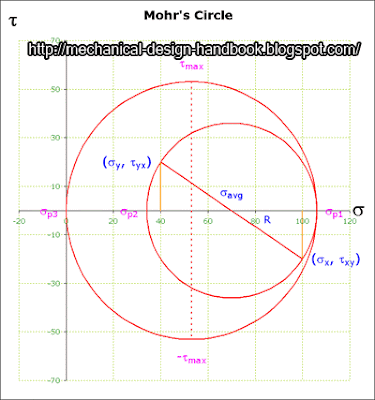## Posts

Showing posts from April, 2008

### Improve math skills of your kids - Learn step-by-step arithmetic from Math games

Math: Unknown - Step-by-step math calculation game for iOS.

Math: Unknown is much more than a math game. It is a step-by-step math calculation game which will teach users how to calculate in the correct order rather than just asking only the final calculated results.

The app consists of four basic arithmetic operations which are addition, subtraction, multiplication and division. In order to get started, users who are new to arithmetic can learn from animated calculation guides showing step-by-step procedures of solving each type of operation. It is also helpful for experienced users as a quick reference.

Generally, addition and subtraction may be difficult for users who just start learning math especially when questions require carrying or borrowing (also called regrouping). The app helps users to visualize the process of carrying and borrowing in the way it will be done on paper. Once users understand how these operations work, they are ready to learn multiplication and division.

For most students, division is considered as the most difficult arithmetic operation to solve. It is a common area of struggle since it requires prior knowledge of both multiplication and subtraction. To help users understand division, the app uses long division to teach all calculation procedures. Relevant multiplication table will be shown beside the question. Users will have to pick a number from the table which go into the dividend. Multiplication of selected number and divisor is automatically calculated, but the users have to do subtraction and drop down the next digit themselves. Learning whole calculation processes will make them master it in no time.

Math: Unknown is a helpful app for students who seriously want to improve arithmetic calculation skills.

### Microsoft Excel VBA - Mohr's Circle for Plane StressThe German engineer Otto Mohr (1835-1918) developed a useful pictorial or graphical interpretation of the equations for finding the principal stresses and the maximum shearing stress at a point in a stressed member. This method, commonly called Mohr's circle, involves the construction of a circle in such a manner that the coordinates of each point on the circle represent the normal and shearing stresses on one plane through the stressed point, and the angular position of the radius to the point gives the orientation of the plane.

Normal stresses are plotted as horizontal coordinates, with tensile stresses (positive) plotted to the right of the origin and compressive stresses (negative) plotted to the left. Shearing stresses are plotted as vertical coordinates, with those tending to produce a clockwise rotation of the stress element plotted above the s axis, and those tending to produce a counterclockwise rotation of the stress element plotted below the s axis.

The results obtaine…